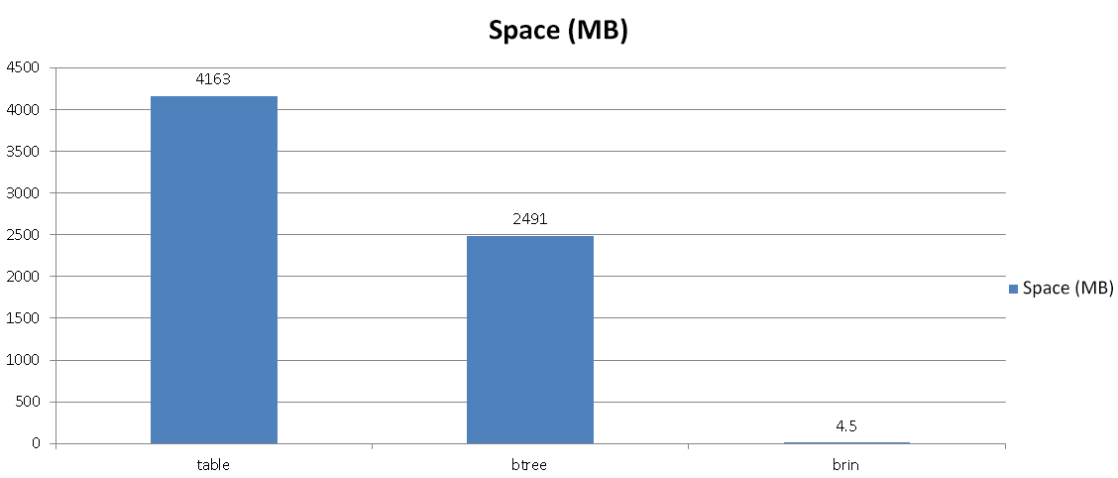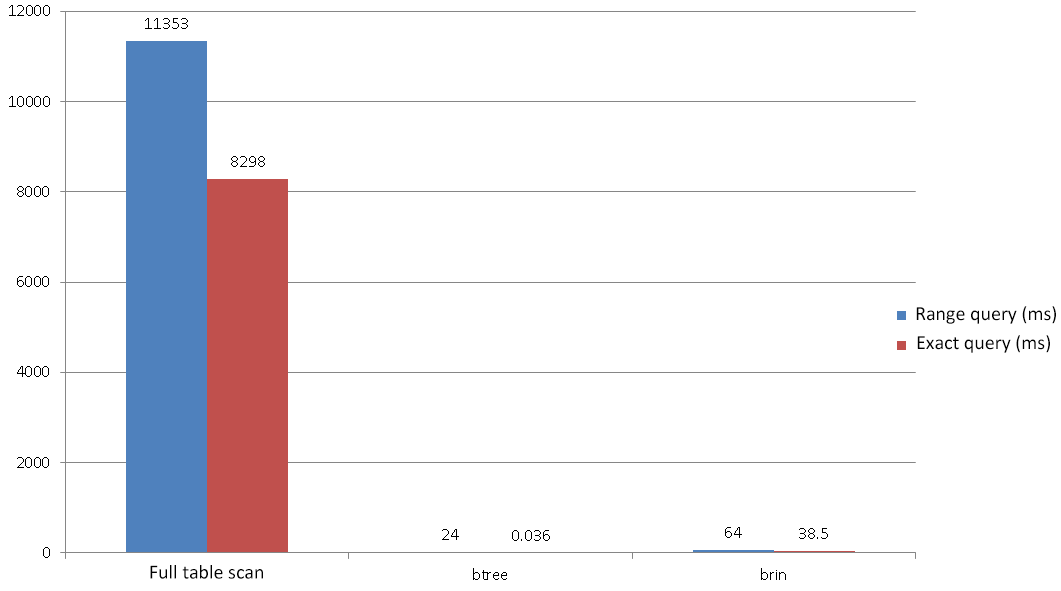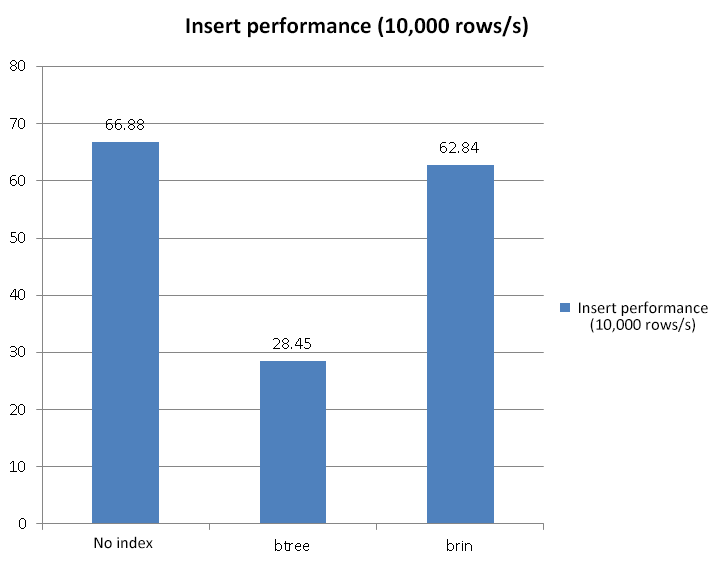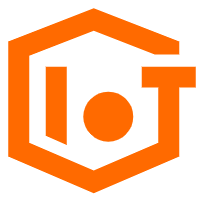# Community

Blog Events Webinars Tutorials Forum
×
Community Blog Create an BRIN Index at a Fraction of the Normal Size

# Create an BRIN Index at a Fraction of the Normal Size

In this blog, we will look into why BTree indexes are not suitable for IoT, and how BRIN indexes are, and how the two of these indexes compare with each other.

By Digoal

The most frequently used database index is BTree. Other common indexes are Hash and BitMap. However, for the Internet of Things (IoT), these indexes are heavy weight and are therefore not suitable because they can lead to significant performance losses.

But why is this the case? Well, IoT generally involves a large amount of data that is generated and stored, akin to data streaming you could say. Well, when you are using this data, the First Input First Output (FIFO) execution method, or the batch data usage style of range query is basically adopted.

In general, because of the contraints of IoT, BRIN indexes are a much better option than BTree indexes. In this blog, we will look into why BTree indexes are not suitable for IoT, and how BRIN indexes are, and how the two of these indexes compare with each other.

## Why BTree Index Is Not Suitable

The BTree index is pretty heavy weight because the index needs to store the value and address the index field of each record, resulting in a huge index. However, because IoT uses a large number of range queries and batch processing, it needs to use a much more light-weight index.

Consider the following example. The BTree index takes up a large proportion of space.

``````postgres=# \dt+ tab
List of relations
Schema | Name | Type  |  Owner   |  Size   | Description
--------+------+-------+----------+---------+-------------
public | tab  | table | postgres | 3438 MB |
(1 row)

postgres=# \di+ idx_tab_id
List of relations
Schema |    Name    | Type  |  Owner   | Table |  Size   | Description
--------+------------+-------+----------+-------+---------+-------------
public | idx_tab_id | index | postgres | tab   | 2125 MB |
(1 row)  ``````

Besides being large in size, BTree indexes also negatively affect the performance of data operations such as updates, deletion, or insertion.

With a BTree index, 284,500 rows are stored per second. Consider the following:

``````postgres=# create unlogged table tab(id serial8, info text, crt_time timestamp);
CREATE TABLE
postgres=# create index idx_tab_id on tab(id);
CREATE INDEX
vi test.sql
insert into tab (info) select '' from generate_series(1,10000);

pgbench -M prepared -n -r -P 1 -f ./test.sql -c 48 -j 48 -T 100
tps = 28.453983 (excluding connections establishing)  ``````

However, without a BTree index, 668,800 rows are stored per second.

``````postgres=# drop index idx_tab_id ;
DROP INDEX
pgbench -M prepared -n -r -P 1 -f ./test.sql -c 48 -j 48 -T 100
tps = 66.880260 (excluding connections establishing)  ``````

From the above introduction and test data, it is clear that the BTree index does negatively affect the performance of other operations.

## How the BRIN Index works

The BRIN index works by storing statistical information of consecutive adjacent blocks (min(val), max(val), has null? all null? left block id, right block id).

For example, assume that a table occupies 10,000 blocks. When creating a BRIN index, statistics for every 128 blocks are specified, then this index only needs to store 79 pieces of statistics. In other words, this index can be useful for IoT because it doesn't occupy much space, unlike other indexes.

## How the BRIN and BTree Indexes Compare

In this section, let's look how these indexes compare in terms of insert performance, as well as size and query performance.

In terms of query performance, with a range index, 628,400 rows are stored per second, which is much higher than that of the BTree index tested in the previous section.

``````postgres=# drop index idx_tab_id ;
DROP INDEX
postgres=# create index idx_tab_id on tab using brin (id) with (pages_per_range=1);
CREATE INDEX
pgbench -M prepared -n -r -P 1 -f ./test.sql -c 48 -j 48 -T 100
tps = 62.838701 (excluding connections establishing)  ``````

Next, let's look at the size and query performance of bolth indexes compared. In terms of size, with the table size being 4163 MB, the size of the BTree index is 2491 MB, and the size of the BRIN index is 4608 KB. Immediately, you can see that the BRIN index is much smaller than the BTree index, despite the relatively large size of the table.

``````postgres=# \di+ idx_tab_btree_id
List of relations
Schema |       Name       | Type  |  Owner   | Table |  Size   | Description
--------+------------------+-------+----------+-------+---------+-------------
public | idx_tab_btree_id | index | postgres | tab   | 2491 MB |
(1 row)

postgres=# \di+ idx_tab_id
List of relations
Schema |    Name    | Type  |  Owner   | Table |  Size   | Description
--------+------------+-------+----------+-------+---------+-------------
public | idx_tab_id | index | postgres | tab   | 4608 kB |
(1 row)

postgres=# \dt+ tab
List of relations
Schema | Name | Type  |  Owner   |  Size   | Description
--------+------+-------+----------+---------+-------------
public | tab  | table | postgres | 4163 MB |
(1 row)  ``````

Next, in terms of query performance, for the range query, the full table scan takes 11 seconds, and the BRIN index takes 64 milliseconds, whereas the BTree index takes 24 milliseconds. So for this, the BTree index is faster than the BRIN index.

``````postgres=# /*+ seqscan(tab) */ explain (analyze,buffers,timing,costs,verbose) select count(*) from tab where id between 1 and 100000;
QUERY PLAN
--------------------------------------------------------------------------------------------------------------------------------
Aggregate  (cost=1891578.12.. 1891578.13 rows=1 width=0) (actual time=11353.057.. 11353.058 rows=1 loops=1)
Output: count(*)
Buffers: shared hit=133202
->  Seq Scan on public.tab  (cost=0.00.. 1891352.00 rows=90447 width=0) (actual time=1660.445.. 11345.123 rows=100000 loops=1)
Output: id, info, crt_time
Filter: ((tab.id >= 1) AND (tab.id <= 100000))
Rows Removed by Filter: 117110000
Buffers: shared hit=133202
Planning time: 0.048 ms
Execution time: 11353.080 ms
(10 rows)

postgres=# /*+ bitmapscan(tab idx_tab_id) */ explain (analyze,buffers,timing,costs,verbose) select count(*) from tab where id between 1 and 100000;
QUERY PLAN
-------------------------------------------------------------------------------------------------------------------------------------
Aggregate  (cost=70172.91.. 70172.92 rows=1 width=0) (actual time=63.735.. 63.735 rows=1 loops=1)
Output: count(*)
Buffers: shared hit=298
->  Bitmap Heap Scan on public.tab  (cost=1067.08.. 69946.79 rows=90447 width=0) (actual time=40.700.. 55.868 rows=100000 loops=1)
Output: id, info, crt_time
Recheck Cond: ((tab.id >= 1) AND (tab.id <= 100000))
Rows Removed by Index Recheck: 893
Heap Blocks: lossy=111
Buffers: shared hit=298
->  Bitmap Index Scan on idx_tab_id  (cost=0.00.. 1044.47 rows=90447 width=0) (actual time=40.675.. 40.675 rows=1110 loops=1)
Index Cond: ((tab.id >= 1) AND (tab.id <= 100000))
Buffers: shared hit=187
Planning time: 0.049 ms
Execution time: 63.755 ms
(14 rows)

postgres=# /*+ bitmapscan(tab idx_tab_btree_id) */ explain (analyze,buffers,timing,costs,verbose) select count(*) from tab where id between 1 and 100000;
QUERY PLAN
--------------------------------------------------------------------------------------------------------------------------------------------
Aggregate  (cost=76817.88.. 76817.89 rows=1 width=0) (actual time=23.780.. 23.780 rows=1 loops=1)
Output: count(*)
Buffers: shared hit=181
->  Bitmap Heap Scan on public.tab  (cost=1118.87.. 76562.16 rows=102286 width=0) (actual time=6.569.. 15.950 rows=100000 loops=1)
Output: id, info, crt_time
Recheck Cond: ((tab.id >= 1) AND (tab.id <= 100000))
Heap Blocks: exact=111
Buffers: shared hit=181
->  Bitmap Index Scan on idx_tab_btree_id  (cost=0.00.. 1093.30 rows=102286 width=0) (actual time=6.530.. 6.530 rows=100000 loops=1)
Index Cond: ((tab.id >= 1) AND (tab.id <= 100000))
Buffers: shared hit=70
Planning time: 0.099 ms
Execution time: 23.798 ms
(13 rows) ``````

Next, for an exact query, the full table scan takes 8 seconds, the BRIN index takes 39 milliseconds, and the BTree index takes 0.03 milliseconds. In other words, and BTree index is still faster than the BRIN index.

``````postgres=# /*+ seqscan(tab) */ explain (analyze,buffers,timing,costs,verbose) select count(*) from tab where id=100000;
QUERY PLAN
----------------------------------------------------------------------------------------------------------------------
Aggregate  (cost=1598327.00.. 1598327.01 rows=1 width=0) (actual time=8297.589.. 8297.589 rows=1 loops=1)
Output: count(*)
Buffers: shared hit=133202
->  Seq Scan on public.tab  (cost=0.00.. 1598327.00 rows=2 width=0) (actual time=1221.359.. 8297.582 rows=1 loops=1)
Output: id, info, crt_time
Filter: (tab.id = 100000)
Rows Removed by Filter: 117209999
Buffers: shared hit=133202
Planning time: 0.113 ms
Execution time: 8297.619 ms
(10 rows)

postgres=# /*+ bitmapscan(tab idx_tab_id) */ explain (analyze,buffers,timing,costs,verbose) select count(*) from tab where id=100000;
QUERY PLAN
------------------------------------------------------------------------------------------------------------------------------
Aggregate  (cost=142.04.. 142.05 rows=1 width=0) (actual time=38.498.. 38.498 rows=1 loops=1)
Output: count(*)
Buffers: shared hit=189
->  Bitmap Heap Scan on public.tab  (cost=140.01.. 142.04 rows=2 width=0) (actual time=38.432.. 38.495 rows=1 loops=1)
Output: id, info, crt_time
Recheck Cond: (tab.id = 100000)
Rows Removed by Index Recheck: 1811
Heap Blocks: lossy=2
Buffers: shared hit=189
->  Bitmap Index Scan on idx_tab_id  (cost=0.00.. 140.01 rows=2 width=0) (actual time=38.321.. 38.321 rows=20 loops=1)
Index Cond: (tab.id = 100000)
Buffers: shared hit=187
Planning time: 0.102 ms
Execution time: 38.531 ms
(14 rows)

postgres=# /*+ indexscan(tab idx_tab_btree_id) */ explain (analyze,buffers,timing,costs,verbose) select count(*) from tab where id=100000;
QUERY PLAN
-----------------------------------------------------------------------------------------------------------------------------------
Aggregate  (cost=2.76.. 2.77 rows=1 width=0) (actual time=0.018.. 0.018 rows=1 loops=1)
Output: count(*)
Buffers: shared hit=4
->  Index Scan using idx_tab_btree_id on public.tab  (cost=0.44.. 2.76 rows=2 width=0) (actual time=0.015.. 0.016 rows=1 loops=1)
Output: id, info, crt_time
Index Cond: (tab.id = 100000)
Buffers: shared hit=4
Planning time: 0.049 ms
Execution time: 0.036 ms
(9 rows)  ``````

## Performance Comparison Charts:## Summary

To sum up the above sections and our findings, the key application scenario of the BRIN index is the range query scenario of IoT, which stores data in a sort of streaming mode. Not only does the range index have minimal impact on insertion, but the index size is small. And, for the range query, the performance difference between the range index and BTree is minimal.

0 0 0
Share on

# Related Products

• ##AnalyticDB for PostgreSQL

An online MPP warehousing service based on the Greenplum Database open source program

• ##IoT Solution

A cloud solution for smart technology providers to quickly build stable, cost-efficient, and reliable ubiquitous platforms

• ##Elastic Block Storage

Block-level data storage attached to ECS instances to achieve high performance, low latency, and high reliability

• ##Link IoT Edge

Link IoT Edge allows for the management of millions of edge nodes by extending the capabilities of the cloud, thus providing users with services at the nearest location.